#### Vol. 34, No. 3, 1970

 Download this articleFor screen For printingRecent Issues Vol. 325: 1  2 Vol. 324: 1  2 Vol. 323: 1  2 Vol. 322: 1  2 Vol. 321: 1  2 Vol. 320: 1  2 Vol. 319: 1  2 Vol. 318: 1  2Online Archive Volume: Issue:The Journal Subscriptions Editorial Board Officers Contacts Submission Guidelines Submission Form Policies for Authors ISSN: 1945-5844 (e-only) ISSN: 0030-8730 (print) Special Issues Author Index To Appear Other MSP Journals
Twisted self-homotopy equivalences

Vol. 34 (1970), No. 3, 789–802
##### Abstract

This paper studies the group G(A × B) of (homotopy classes of) self-homotopy equivalences of a product A × B of two connected CW homotopy associative H-spaces A and B. It establishes the existence of an exact sequence of multiplicative groups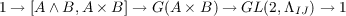provided that i[A×B,A×B] q [AB,A×B] = 0, where q : A×B AB is the cofibration induced by the inclusion i : A B A × B of the sum into the product. The entry GL(2,ΛIJ) is the group of invertible matrices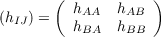with entries hIJ in the homotopy sets ΛIJ = [I,J] for I,J = A,B, where matrix multiplication is defined by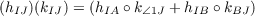in terms of composition and the operation + in the homotopy sets [I,J], and where the multiplicative unit is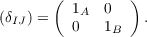The homomorphism G(A × B) GL(2,ΛIiI) is given by the correspondence of h : A × B A × B with the matrix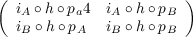with entries obtained from h by composing with the inclusions iA : A A×B and iB : B A × B and the projections pA : A × B A and pB : A × B B; for a preliminary result states that under the hypothesis above h : A × B A × B is a homotopy equivalence if and only if the matrix (iI h pJ) is invertible.

A homotopy equivalence f ×g : A×B A×B is referred to as untwisted. These determine a subgroup G(A) × G(B) G(A × B) which is isomorphic under the homomorphism G(A×B) GL(2,ΛIJ) to the subgroup of diagonal matrices, and so the nondiagonal matrices give measure of the twisted selfhomotopy equivalences A × B A × B. The extreme case in which all self-homotopy equivalences are untwisted is considered, and it is shown that G(A) ×G(B) = G(A×B) if and only if the homotopy sets [A,B], [B,A], and [A B,A × B] are trivial.

Next, four settings are considered in which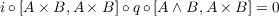and the exact sequence is valid. In the last section the dual situation of the group G(M N) of self-homotopy equivalences of a sum M N of two co-H-spaces M and N is briefly sketched.

Primary: 55.40
##### Milestones